# Time variant system reliability problem¶

The objective is to evaluate the outcrossing rate from a safe to a failure domain in a time variant reliability problem.

We consider the following limit state function, defined as the difference between a degrading resistance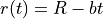and a time-varying load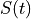:

The failure domaine is defined by: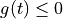which means that the resistance atis less than the stress at.

We propose the following probabilistic model:

•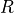is the initial resistance, and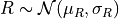;

•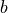is the deterioration rate of the resistance; it is deterministic;

•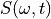is the time-varying stress, which is modeled by a stationary Gaussian process of mean value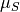, standard deviation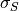and a squared exponential covariance model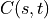.

The outcrossing rate from the safe to the failure domain at instantis defined by: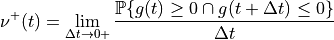For each, we note the random variable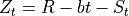where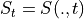.

To evaluate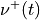, we need to consider the bivariate random vector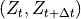.

The event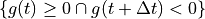writes as the intersection of both events :

•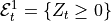and

•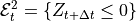.

The objective is to evaluate: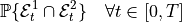## 1. Define some useful functions¶

We define the bivariate random vector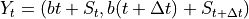. Here,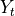is a bivariate Normal random vector:

• with mean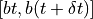and

• with covariance matrix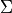defined by: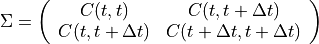This function buils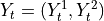.

from math import sqrt
from openturns.viewer import View
import openturns as ot

def buildNormal(b, t, mu_S, covariance, delta_t=1e-5):
sigma = ot.CovarianceMatrix(2)
sigma[0, 0] = covariance(t, t)[0, 0]
sigma[0, 1] = covariance(t, t + delta_t)[0, 0]
sigma[1, 1] = covariance(t + delta_t, t + delta_t)[0, 0]
return ot.Normal([b * t + mu_S, b * (t + delta_t) + mu_S], sigma)


This function creates the trivariate random vector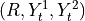whereis independent from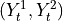. We need to create this random vector because both events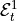and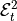must be defined from the same random vector!

def buildCrossing(b, t, mu_S, covariance, R, delta_t=1e-5):
normal = buildNormal(b, t, mu_S, covariance, delta_t)
return ot.BlockIndependentDistribution([R, normal])


This function evaluates the probability using the Monte Carlo sampling. It defines the intersection event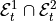.

def getXEvent(b, t, mu_S, covariance, R, delta_t):
full = buildCrossing(b, t, mu_S, covariance, R, delta_t)
X = ot.RandomVector(full)
f1 = ot.SymbolicFunction(["R", "X1", "X2"], ["X1 - R"])
e1 = ot.ThresholdEvent(ot.CompositeRandomVector(f1, X), ot.Less(), 0.0)
f2 = ot.SymbolicFunction(["R", "X1", "X2"], ["X2 - R"])
e2 = ot.ThresholdEvent(ot.CompositeRandomVector(f2, X), ot.GreaterOrEqual(), 0.0)
event = ot.IntersectionEvent([e1, e2])
return X, event

def computeCrossingProbability_MonteCarlo(
b, t, mu_S, covariance, R, delta_t, n_block, n_iter, CoV
):
X, event = getXEvent(b, t, mu_S, covariance, R, delta_t)
algo = ot.ProbabilitySimulationAlgorithm(event, ot.MonteCarloExperiment())
algo.setBlockSize(n_block)
algo.setMaximumOuterSampling(n_iter)
algo.setMaximumCoefficientOfVariation(CoV)
algo.run()
return algo.getResult().getProbabilityEstimate() / delta_t


This function evaluates the probability using the Low Discrepancy sampling.

def computeCrossingProbability_QMC(
b, t, mu_S, covariance, R, delta_t, n_block, n_iter, CoV
):
X, event = getXEvent(b, t, mu_S, covariance, R, delta_t)
algo = ot.ProbabilitySimulationAlgorithm(
event,
ot.LowDiscrepancyExperiment(ot.SobolSequence(X.getDimension()), n_block, False),
)
algo.setBlockSize(n_block)
algo.setMaximumOuterSampling(n_iter)
algo.setMaximumCoefficientOfVariation(CoV)
algo.run()
return algo.getResult().getProbabilityEstimate() / delta_t


This function evaluates the probability using the FORM algorithm for event systems..

def computeCrossingProbability_FORM(b, t, mu_S, covariance, R, delta_t):
X, event = getXEvent(b, t, mu_S, covariance, R, delta_t)
algo = ot.SystemFORM(ot.SQP(), event, X.getMean())
algo.run()
return algo.getResult().getEventProbability() / delta_t


## 2. Evaluate the probability¶

First, fix some parameters: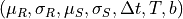and the covariance model which is the Squared Exponential model. Be careful to the parameter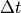which is of great importance: if it is too small, the simulation methods have problems to converge because the correlation rate is too high between the instantsand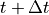. We advice to take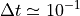.

mu_S = 3.0
sigma_S = 0.5
ll = 10

b = 0.01

mu_R = 5.0
sigma_R = 0.3
R = ot.Normal(mu_R, sigma_R)

covariance = ot.SquaredExponential([ll / sqrt(2)], [sigma_S])

t0 = 0.0
t1 = 50.0
N = 26

# Get all the time steps t
times = ot.RegularGrid(t0, (t1 - t0) / (N - 1.0), N).getVertices()

delta_t = 1e-1


Use all the methods previously described:

• Monte Carlo: values in values_MC

• Low discrepancy suites: values in values_QMC

• FORM: values in values_FORM

values_MC = list()
values_QMC = list()
values_FORM = list()

for tick in times:
values_MC.append(
computeCrossingProbability_MonteCarlo(
b, tick, mu_S, covariance, R, delta_t, 2 ** 12, 2 ** 3, 1e-2
)
)
values_QMC.append(
computeCrossingProbability_QMC(
b, tick, mu_S, covariance, R, delta_t, 2 ** 12, 2 ** 3, 1e-2
)
)
values_FORM.append(
computeCrossingProbability_FORM(b, tick, mu_S, covariance, R, delta_t)
)

print("Values MC = ", values_MC)
print("Values QMC = ", values_QMC)
print("Values FORM = ", values_FORM)

Values MC =  [0.0, 0.0, 0.0, 0.0, 0.0, 0.0, 0.00030517578125, 0.0, 0.0006103515625, 0.0, 0.0, 0.0, 0.0006103515625, 0.0, 0.0006103515625, 0.0006103515625, 0.00030517578125, 0.00030517578125, 0.0, 0.0006103515625, 0.0006103515625, 0.00030517578125, 0.001220703125, 0.00091552734375, 0.0006103515625, 0.001220703125]
Values QMC =  [0.0, 0.0, 0.0, 0.0, 0.0, 0.0, 0.0006103515625, 0.0, 0.0006103515625, 0.0, 0.0, 0.0, 0.00030517578125, 0.00030517578125, 0.0, 0.00030517578125, 0.0, 0.00091552734375, 0.0006103515625, 0.0, 0.00030517578125, 0.0, 0.0006103515625, 0.001220703125, 0.00091552734375, 0.0006103515625]
Values FORM =  [6.407247221976507e-05, 7.202731340749856e-05, 8.087457586090277e-05, 9.070184981054664e-05, 0.00010160352566970394, 0.00011368175073412876, 0.0001270463113912958, 0.00014181491000870818, 0.00015811435561607578, 0.00017607979215241996, 0.00019585595886454746, 0.0002175971126689752, 0.0002414674407523497, 0.0002676410518535999, 0.0002963031343688264, 0.0003276489835870634, 0.00036188514225255717, 0.00039922842206094566, 0.0004399070455775222, 0.000484160922259269, 0.0005322401306923784, 0.0005844062178174964, 0.0006409303353549688, 0.000702094564935671, 0.0007681919118323028, 0.0008395236033398269]


Draw the graphs!

g = ot.Graph()
g.setAxes(True)
g.setGrid(True)
c = ot.Curve(times, [[p] for p in values_MC])